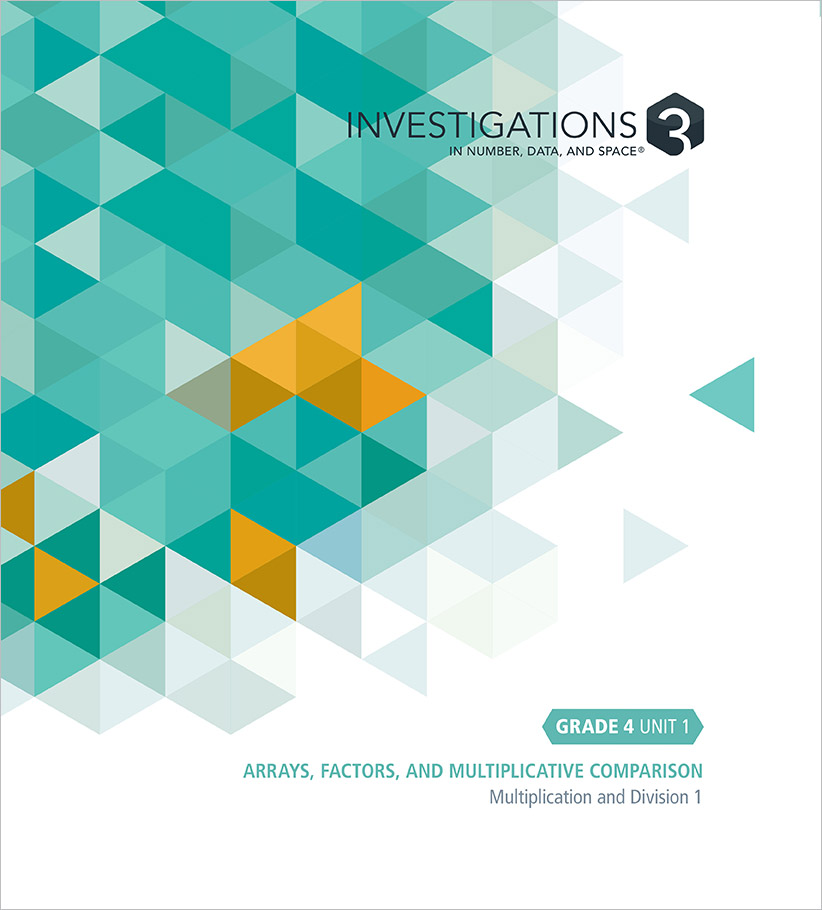Select Page

#### Unit 1: Arrays, Factors, and Multiplicative Comparison (12 Sessions)

##### Multiplication and Division 1

This unit focuses on using arrays and multiplicative comparison problems to understand multiplication, and gaining familiarity with factors and multiples. Students use arrays to model multiplication situations and to find factors and identify prime numbers to 100. They use what they know about factors of 100 to find factors of multiples of 100, and examine the idea that factors of a number are also factors of a multiple of that number.

• Investigation 1: Representing Multiplication with Arrays (8 Sessions)
• Investigation 2: Finding Factors (4 Sessions)

#### Unit 2: Generating and Representing Measurement Data (11 Sessions)

##### Modeling with Data

This unit focuses on using line plots to represent, describe, and compare measurement data; on modeling real-world problems with mathematics; and on constructing arguments based on data. Students measure and compare the heights of first and fourth graders. They collect measurement data of their choosing, and use line plots to represent and analyze the data.

• Investigation 1: Modeling with Data (5 Sessions)
• Investigation 2: Using Data to Compare (6 Sessions)

#### Unit 3: Multiple Towers and Cluster Problems (18 Sessions)

##### Multiplication and Division 2

This unit focuses on solving multiplication problems with 2-digit numbers, understanding the meaning and structure of, and the relationship between, multiplication and division, and using that understanding to solve multiplication and division problems. Students use marked and unmarked arrays to represent multiplication strategies that involve breaking numbers apart. They solve and represent division story problems, including problems with remainders. Students develop strategies for breaking the numbers in larger multiplication problems apart in order to use number relationships that they know, including multiplying by a multiple of 10.

• Investigation 1: Breaking Apart Multiplication Problems (5 Sessions)
• Investigation 2: Solving Division Problems (6 Sessions)
• Investigation 3: Strategies for Multiplication (7 Sessions)

#### Unit 4: Measuring and Classifying Shapes (20 Sessions)

##### 2-D Geometry and Measurement

This unit focuses on measuring with standard units, including perimeter, area, and angles; describing and classifying 2-D shapes, and identifying mirror symmetry.

• Investigation 1: Linear Measurement (5 Sessions)
• Investigation 2: Sorting and Classifying Polygons (5 Sessions)
• Investigation 3: Measuring Angles (4 Sessions)
• Investigation 4: Symmetry and Area (6 Sessions)

#### Unit 5: Large Numbers and Landmarks (19 Sessions)

##### Addition, Subtraction, and the Number System

This unit focuses on understanding the meaning of addition and subtraction, understanding the base-10 number system with numbers to 1,000,000, and adding and subtracting multidigit numbers fluently, including with the U.S. standard algorithms.

• Investigation 1: Studying Addition (6 Sessions)
• Investigation 2: Studying Subtraction (7 Sessions)
• Investigation 3: Adding and Subtracting Large Numbers (6 Sessions)

#### Unit 6: Fraction Cards and Decimal Grids (24 Sessions)

##### Fractions and Decimals

This unit focuses on understanding the meaning of fractions and decimals; and comparing fractions and decimals including finding equivalents. Students use contexts and representations such as rectangles (an area model) and number lines (a linear model) to add, subtract, and multiply fractions.

• Investigation 1: Parts of Rectangles (6 Sessions)
• Investigation 2: Comparing Fractions and Decimals (8 Sessions)
• Investigation 3: Adding and Subtracting Fractions and Decimals (6 Sessions)
• Investigation 4: Computation with Rational Numbers (4 Sessions)

#### Unit 7: How Many Packages and Groups? (18 Sessions)

##### Multiplication and Division 3

This unit focuses on the operations of multiplication and division, including problems involving converting measurements. Students refine their strategies for solving multiplication problems with two 2-digit numbers and with a 4-digit number and a 1-digit number, and they use the relationship between multiplication and division to develop and practice strategies for solving division problems with up to a 4-digit dividend and a 1-digit divisor.

• Investigation 1: Multiplication with 2-Digit Numbers (7 Sessions)
• Investigation 2: Strategies for Multiplication (5 Sessions)
• Investigation 3: Solving Division Problems (6 Sessions)

#### Unit 8: Penny Jars and Towers (10 Sessions)

##### Analyzing Patterns and Rules

This unit focuses on solving multi-step problems, generating and analyzing patterns, and using symbolic notation to model situations. Two contexts (Penny Jars and Windows and Towers) are used that include both additive and multiplicative situations.

• Investigation 1: Representing and Analyzing Patterns (10 Sessions)